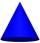# Cuboid edges in ratio

Cuboid edges lengths are in ratio 2:4:6. Calculate their lengths if you know that the cuboid volume is 24576 cm3.

Result

a =  16 cm
b =  32 cm
c =  48 cm

#### Solution:Leave us a comment of example and its solution (i.e. if it is still somewhat unclear...):

Showing 0 comments:Be the first to comment!#### To solve this example are needed these knowledge from mathematics:

Tip: Our volume units converter will help you with converion of volume units.

## Next similar examples:

1. Max - coneFrom the iron bar (shape = prism) with dimensions 6.2 cm, 10 cm, 6.2 cm must be produced the greatest cone. a) Calculate cone volume. b) Calculate the waste.
2. RainIt rains at night. On 1 m2 of lake will drop 60 liters of water. How many cm will the lake level rise?
3. Swimming poolThe pool shape of cuboid is 299 m3 full of water. Determine the dimensions of its bottom if water depth is 282 cm and one bottom dimension is 4.7 m greater than the second.
4. Prism basesVolume perpendicular quadrilateral prism is 360 cm3. The edges of the base and height of the prism are in the ratio 5:4:2 Determine the area of the base and walls of the prism.
5. Cube cornersFrom cube of edge 14 cm cut off all vertices so that each cutting plane intersects the edges 1 cm from the nearest vertice. How many edges will have this body?
6. Two boxes-cubesTwo boxes cube with edges a=38 cm and b = 81 cm is to be replaced by one cube-shaped box (same overall volume). How long will be its edge?
7. Scale factorA prism with a volume of 1458 mm3 is scaled down to a volume of 16mm3. What is the scale factor in fraction form?
8. Cube 9What was the original edge length of the cube if after cutting 39 small cubes with an edge length 2 dm left 200 dm3?
9. The volume 2The volume of a cube is 27 cubic meters. Find the height of the cube.
10. TetrahedronCalculate height and volume of a regular tetrahedron whose edge has a length 18 cm.
11. Truncated coneCalculate the height of the rotating truncated cone with volume V = 1115 cm3 and a base radii r1 = 7.9 cm and r2 = 9.7 cm.
12. BottlesThe must is sold in 5-liter and 2-liter bottles. Mr Kucera bought a total of 216 liters in 60 bottles. How many liters did Mr. Kucera buy in five-liter bottles?
13. Theorem proveWe want to prove the sentense: If the natural number n is divisible by six, then n is divisible by three. From what assumption we started?
14. Holidays - on poolChildren's tickets to the swimming pool stands x € for an adult is € 2 more expensive. There was m children in the swimming pool and adults three times less. How many euros make treasurer for pool entry?
15. Expression with powersIf x-1/x=5, find the value of x4+1/x4
16. AlgebraX+y=5, find xy (find the product of x and y if x+y = 5)
17. CoefficientDetermine the coefficient of this sequence: 7.2; 2.4; 0.8